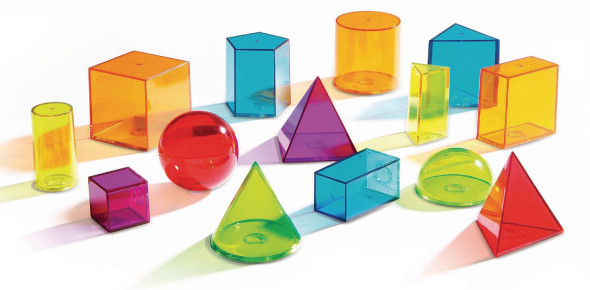# Geometry: Segment Questions! Trivia Quiz

39 Questions | Attempts: 3650
ShareSettingsBelow is a geometry trivia quiz made of segment questions! There are a lot of people who get confused when it comes to segments and the quiz below is designed to help them with the basics but also help them solve problems involving segments. How about you give it a try and get to see how much practice you might need to perfectly understand the topic. Keep an eye out for more questions like it!

• 1.
a) faces _______________                                                                                    b) bases ____________                                                                                     c)  edges _____________                                                                                    d)  vertices ______________
• A.

A:15 B:9 C:8 D:1

• B.

A:7 B:2 C:12 D:8

• C.

A:6 B:2 C:12 D:8

• D.

A:6 B:2 C:12 D:7

• 2.
A) faces ____________                                                                     b)bases ____________                                                                     c) edges ____________                                                                     d) vertices ___________
• A.

A:6 B:2 C:9 D:6

• B.

A:7 B:12 C:8 D:1

• C.

A:5 B:2 C:8 D:6

• D.

A:5 B:2 C:9 D:6

• 3.
3 vertices =
• A.

Triangle

• B.

• C.

Pentagon

• D.

Hexagon

• 4.
4 vertices =
• A.

Pentagon

• B.

Octagon

• C.

• D.

Decagon

• 5.
10 vertices=
• A.

Octagon

• B.

Decagon

• C.

Rectagon

• D.

Nonegon

• 6.
8 vertices =
• A.

Decagon

• B.

Triangle

• C.

Heptagon

• D.

Octagon

• 7.
5 vertices=
• A.

Pentagon

• B.

Decagon

• C.

Nonegon

• D.

Triangle

• 8.
7 vertices
• A.

Nonegon

• B.

Heptagon

• C.

Decagon

• D.

Octogon

• 9.
6 vertices
• A.

Pentagon

• B.

• C.

Hexagon

• D.

Right angle

• 10.
Is it true that a complementary angle is two angles that add up to 90 degrees
• A.

True

• B.

False

• 11.
Is it true that a supplementary angle is two angles that add up to 180 degrees
• A.

Yes

• B.

No

• 12.
What are the proper names for these 2 figures?
• A.

Line AB and line GH

• B.

Line segment AB and line segment GH

• C.

• 13.
What is a 4 sided shape called?
• A.

Triangle

• B.

• C.

Pentagon

• 14.
What tranformation happened if a shape was flipped?
• A.

Rotation

• B.

Reflection

• C.

Translation

• 15.
On a coordinate plane, what is the point called where the two axes meet?
• A.

X-axis

• B.

Y-axis

• C.

Origin

• 16.
A triangle that has no equal sides and no equal angles is called what?
• A.

Equilateral

• B.

Iscoceles

• C.

Scalene

• 17.
Which of the following is an undefined term of geometry?
• A.

Point

• B.

Linear pair

• C.

Vertical angles

• 18.
A line pair is a pair of angles that sum to ____ degrees
• A.

90

• B.

60

• C.

180

• 19.
Two angles are ____ if they have the same measure.
• A.

Linear

• B.

Congruent

• C.

Supplementary

• 20.
Two angles are supplementary if they sum to 90 degrees.
• A.

True

• B.

False

• 21.
Two angles are complementary if they sum of 90 degrees.
• A.

True

• B.

False

• 22.
Which of the following is an undefined term of geometry?
• A.

Point

• B.

Linear pair

• C.

Vertical angles

• 23.
A line pair is a pair of angles that sum to ____ degrees
• A.

90

• B.

60

• C.

180

• 24.
Two angles are ____ if they have the same measure.
• A.

Linear

• B.

Congruent

• C.

Supplementary

• 25.
Two angles are supplementary if they sum to 90 degrees.
• A.

True

• B.

False

## Related TopicsBack to top
×

Wait!
Here's an interesting quiz for you.